# Verbal Reasoning - Character Puzzles

### Exercise :: Character Puzzles - Character Puzzles 1

26.

Which one will replace the question mark ?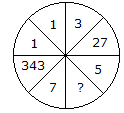A. 25 B. 625 C. 125 D. 50

Explanation:

All numbers are cubed,

(7)3 = 343

(1)3 = 1

(3)3 = 27

Similarly, (5)3 = 125.

27.

Which one will replace the question mark ?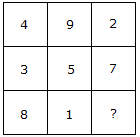A. 9 B. 6 C. 15 D. 14

Explanation:

(4 + 9 + 2) = (3 + 5 + 7) = (8 + 1 + 6)

Total in each case = 15.

28.

Which one will replace the question mark ?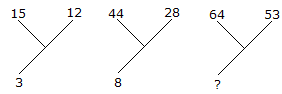A. 30 B. 13 C. 70 D. 118

Explanation:

(15 + 12)/9 = 3

and (44 + 28)/9 = 8

Therefore, (64 + 53)/9 = 13.

29.

Which one will replace the question mark ?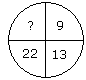A. 40 B. 38 C. 44 D. 39

Explanation:

9 + (2)2 = 13

13 + (3)2 = 22

22 + (4)2 = 38.

30.

Which one will replace the question mark ?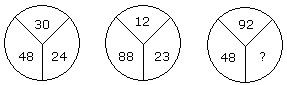A. 60 B. 46 C. 86 D. 75

Explanation:

(30 - 24) x 8 = 48

and (23 - 12) x 8 = 88

Therefore, (92 - 86) x 8 = 48.